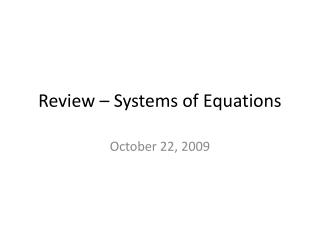DownloadDownload PresentationReview – Systems of Equations

# Review – Systems of Equations

Download Presentation## Review – Systems of Equations

- - - - - - - - - - - - - - - - - - - - - - - - - - - E N D - - - - - - - - - - - - - - - - - - - - - - - - - - -
##### Presentation Transcript

1. Review – Systems of Equations October 22, 2009

2. Objectives Content Objectives Review solving systems of equations. Language Objectives Everyone participates appropriately.

3. Ways to Solve Systems of Equations • Graphically • Algebraically • Addition/elimination method • Substitution method

4. 1. In a class of 35 pupils there are 7 more girls than boys. How many are there of each?

5. 3. The sum of the ages of two brothers is 43 years, and one of them is 15 years older than the other. Find their ages.

6. 4. Divide the number 70 into two parts, such that one part shall be 26 less than the other part.

7. 5. John and Henry together have 143 marbles. If I should give Henry 15 more, he would have just as many asJohn. How many has each?

8. 6. In a storehouse containing 57 barrels there are 3 less barrels of flour than of meal. How many of each?

9. 7. A man whose herd of cows numbered 63 had 17 more Jerseys than Holsteins. How many had he of each?

10. 8. Two men whose wages differ by 8 dollars receive both together \$44 per month. How much does eachreceive?

11. 9. At an election in which 1079 votes were cast the successful candidate had a majority of 95. How many votes did each of the two candidates receive?

12. 10. The sum of three numbers is 56; the second is 3 more than the first and the third 5 more than the first.What are the numbers?

13. 11. Divide 62 into three parts such that the first part is 4 more than the second and the third 7 more than thesecond.

14. 12. Three men together received \$34,200; if the second received \$1500 more than the first, and the third \$1200 more than the second, how much did each receive?

15. 13. Divide 65 into three parts such that the second part is 17 more than the first part, and the third 15 less thanthe first.

16. 14. A man had 95 sheep in three flocks. In the first flock there were 23 more than in the second, and in the third flock 12 less than in the second. How many sheep in each flock?

17. 15. In an election, in which 1073 ballots were cast, Mr. A receives 97 votes less than Mr. B, and Mr. C 120 votes more than Mr. B. How many votes did each receive?

18. 16. A man owns three farms. In the first there are 5 acres more than in the second and 7 acres less than in the third. If there are 53 acres in all the farms together, how many acres are there in each farm?

19. 17. Divide 111 into three parts so that the first part shall be 16 more than the second and 19 less than the third.

20. 18. Three firms lost \$118,000 by fire. The second firm lost \$6000 less than the first and \$20,000 more than thethird. What was each firm's loss?